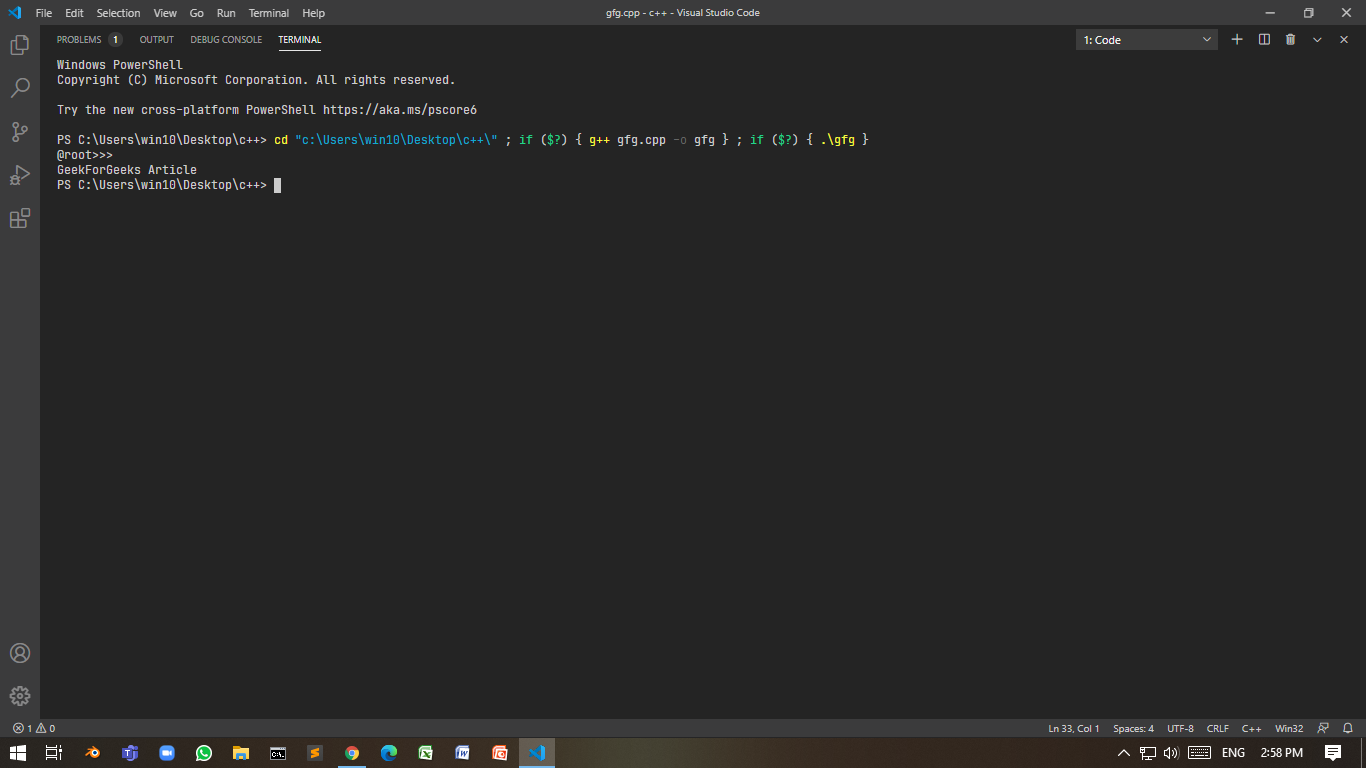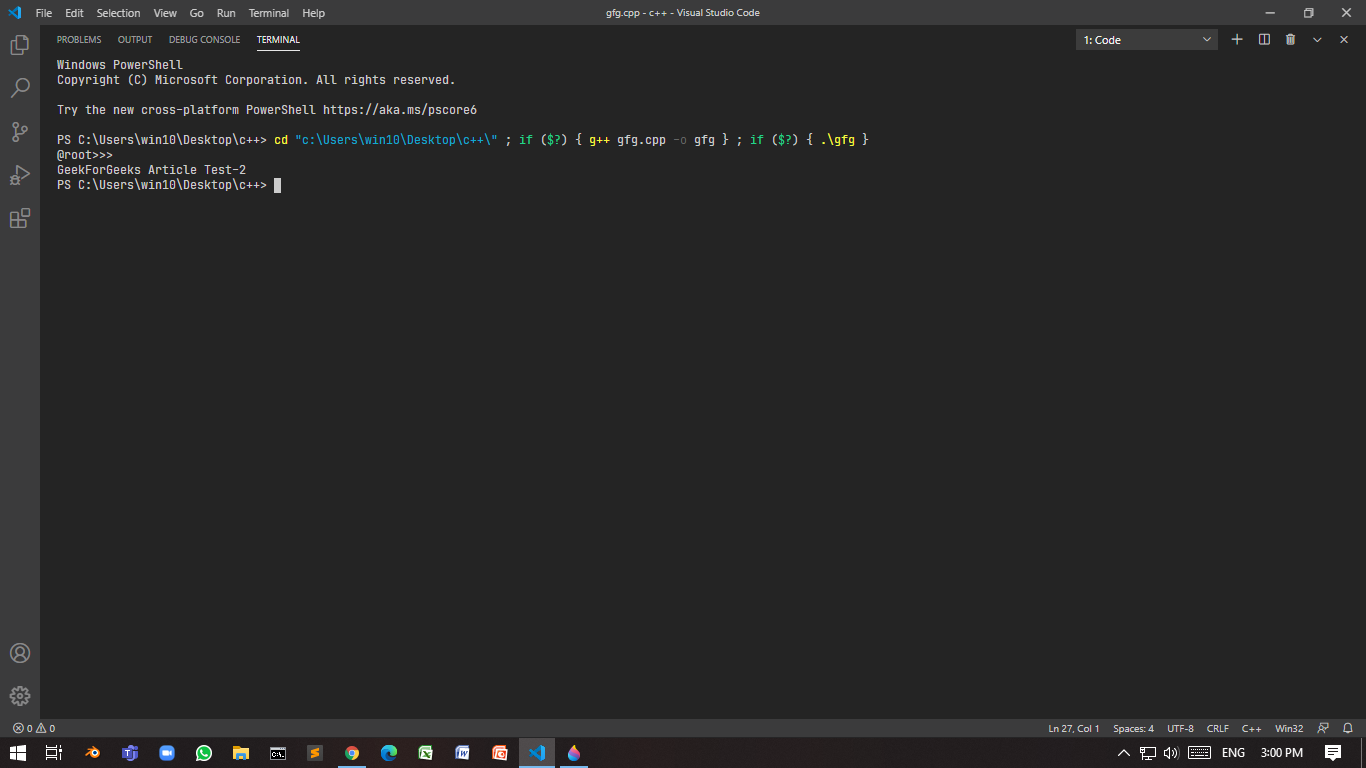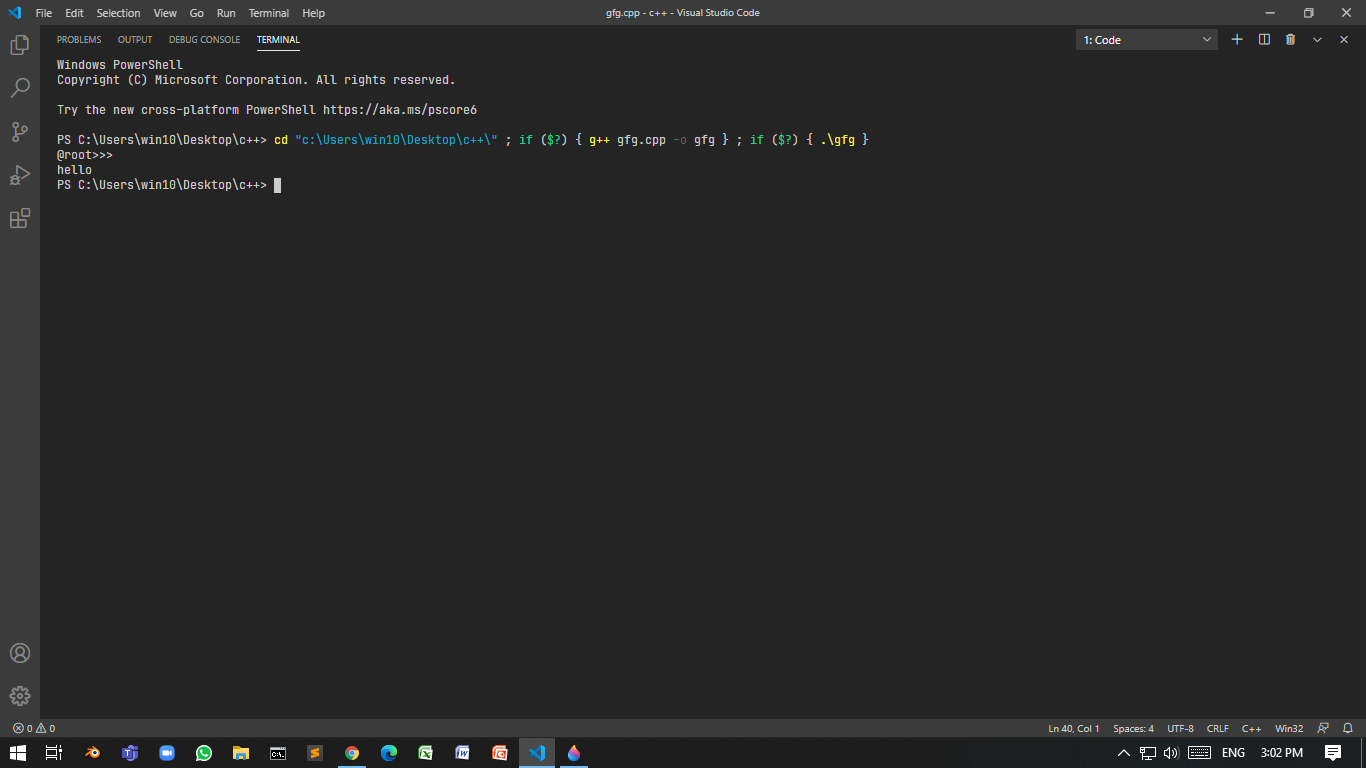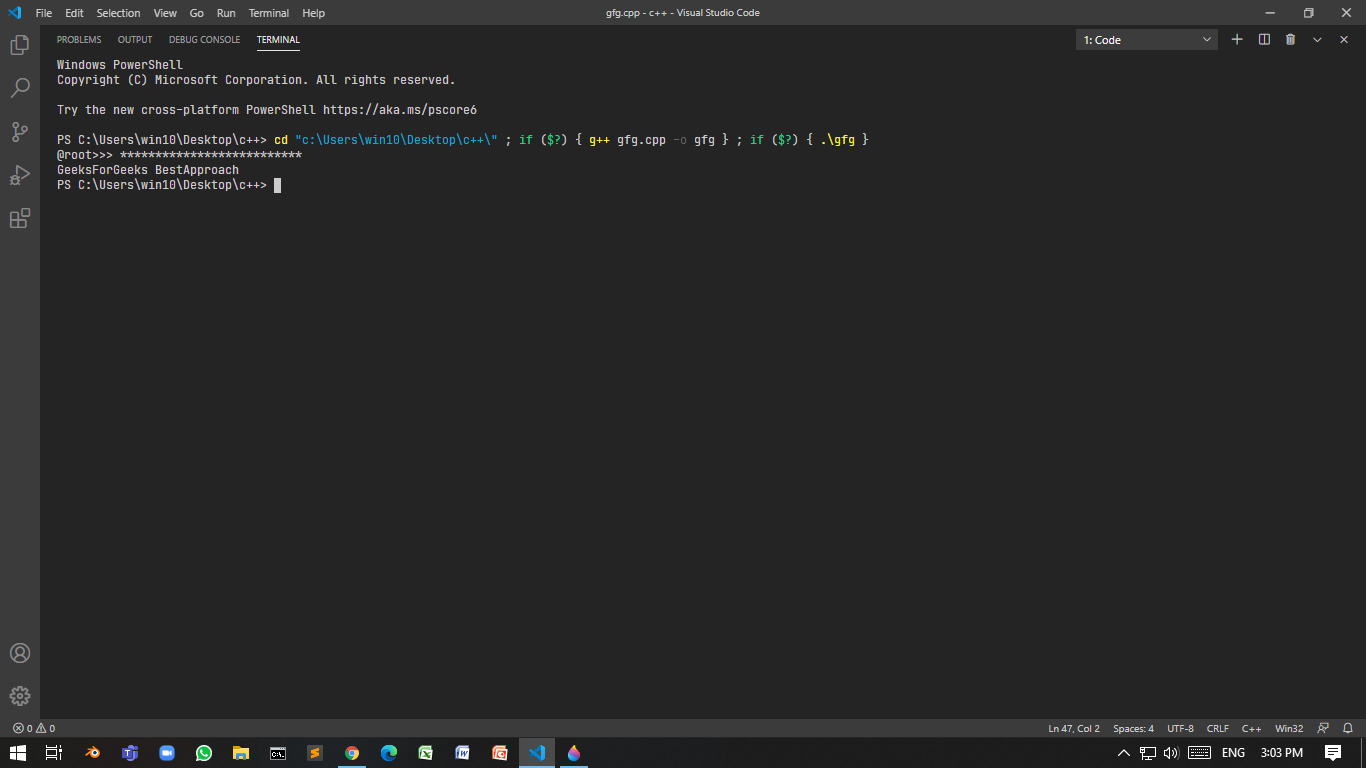# Taking password as input in C++

• Difficulty Level : Hard
• Last Updated : 21 Aug, 2021

There are two methods in which input can be taken in a more secure way:

• Do not display any content.
• Display a special character such as an asterisk instead of actual content.

In this method, input content will be invisible. This can be implemented in two ways:

### using <windows.h>:

Program 1:

Below is the program where console mode is set to enable, echo input, and reset the console mode:

## C++

 `// C++ program to take the input` `// invisibly` `#include ` `#include ` `using` `namespace` `std;`   `// Function take password and` `// reset to console mode` `std::string takePasswdFromUser()` `{` `    ``HANDLE` `hStdInput` `        ``= GetStdHandle(STD_INPUT_HANDLE);` `    ``DWORD` `mode = 0;`   `    ``// Create a restore point Mode` `    ``// is know 503` `    ``GetConsoleMode(hStdInput, &mode);`   `    ``// Enable echo input` `    ``// set to 499` `    ``SetConsoleMode(` `        ``hStdInput,` `        ``mode & (~ENABLE_ECHO_INPUT));`   `    ``// Take input` `    ``string ipt;` `    ``getline(cin, ipt);`   `    ``// Otherwise next cout will print` `    ``// into the same line` `    ``cout << endl;`   `    ``// Restore the mode` `    ``SetConsoleMode(hStdInput, mode);`   `    ``return` `ipt;` `}`   `// Driver Code` `int` `main()` `{` `    ``string input;` `    ``cout << ``"@root>>> "``;`   `    ``// Function Call` `    ``input = takePasswdFromUser();`   `    ``// Print the input` `    ``cout << input << endl;` `}`

Output:### using <conio.h>:

For this getch() is used. This function takes a character input from user without buffer and doesn’t wait for the user to press “return” key.

Program 2:

Below is the C++ program to demonstrate the use of getch() in conio.h:

## C++

 `// C++ program to demonstrate the` `// use of getch()` `#include ` `#include ` `using` `namespace` `std;`   `// Function using getch()` `std::string takePasswdFromUser()` `{` `    ``string ipt = ``""``;` `    ``char` `ipt_ch;` `    ``while` `(``true``) {` `        ``ipt_ch = getch();`   `        ``// Check whether user enters` `        ``// a special non-printable` `        ``// character` `        ``if` `(ipt_ch < 32) {` `            ``cout << endl;` `            ``return` `ipt;` `        ``}` `        ``ipt.push_back(ipt_ch);` `    ``}` `}`   `// Driver Code` `int` `main()` `{` `    ``string input;` `    ``cout << ``"@root>>> "``;`   `    ``// Function call` `    ``input = takePasswdFromUser();` `    ``cout << input << endl;` `}`

Output:Drawback: The user can’t clear the response made earlier. When backspace is pressed, the input is returned.

Program 3:

Below is the C++ program to demonstrate the solution to the above drawback:

## C++

 `// C++ program to demonstrate the` `// solution of above drawback` `#include ` `#include ` `using` `namespace` `std;`   `// Enumerator` `enum` `TT_Input {`   `    ``// ASCII code of backspace is 8` `    ``BACKSPACE = 8,` `    ``RETURN = 32` `};`   `// Function accepting password` `std::string takePasswdFromUser()` `{` `    ``string ipt = ``""``;` `    ``char` `ipt_ch;` `    ``while` `(``true``) {` `        ``ipt_ch = getch();`   `        ``if` `(ipt_ch < TT_Input::RETURN` `            ``&& ipt_ch != TT_Input::BACKSPACE) {` `            ``cout << endl;` `            ``return` `ipt;` `        ``}`   `        ``// Check whether the user` `        ``// pressed backspace` `        ``if` `(ipt_ch == TT_Input::BACKSPACE) {`   `            ``// Check if ipt is empty or not` `            ``if` `(ipt.length() == 0)` `                ``continue``;` `            ``else` `{`   `                ``// Removes last character` `                ``ipt.pop_back();`   `                ``continue``;` `            ``}` `        ``}` `        ``ipt.push_back(ipt_ch);` `    ``}` `}`   `// Driver Code` `int` `main()` `{` `    ``string input;` `    ``cout << ``"@root>>> "``;`   `    ``// Function call` `    ``input = takePasswdFromUser();` `    ``cout << input << endl;` `}`### Hiding the password by a special character(*):

The idea is to use the library <conio.h> here to hide password with asterisk(*). Below is the C++ program using conio.h to hide the password using *:

Program 4:

## C++

 `// C++ program to hide the password` `// using *(asterik)` `#include ` `#include ` `using` `namespace` `std;`   `// Enumerator` `enum` `IN {`   `    ``// 13 is ASCII for carriage` `    ``// return` `    ``IN_BACK = 8,` `    ``IN_RET = 13`   `};`   `// Function that accepts the password` `std::string takePasswdFromUser(` `    ``char` `sp = ``'*'``)` `{` `    ``// Stores the password` `    ``string passwd = ``""``;` `    ``char` `ch_ipt;`   `    ``// Until condition is true` `    ``while` `(``true``) {`   `        ``ch_ipt = getch();`   `        ``// if the ch_ipt` `        ``if` `(ch_ipt == IN::IN_RET) {` `            ``cout << endl;` `            ``return` `passwd;` `        ``}` `        ``else` `if` `(ch_ipt == IN::IN_BACK` `                 ``&& passwd.length() != 0) {` `            ``passwd.pop_back();`   `            ``// Cout statement is very` `            ``// important as it will erase` `            ``// previously printed character` `            ``cout << ``"\b \b"``;`   `            ``continue``;` `        ``}`   `        ``// Without using this, program` `        ``// will crash as \b can't be` `        ``// print in beginning of line` `        ``else` `if` `(ch_ipt == IN::IN_BACK` `                 ``&& passwd.length() == 0) {` `            ``continue``;` `        ``}`   `        ``passwd.push_back(ch_ipt);` `        ``cout << sp;` `    ``}` `}`   `// Driver Code` `int` `main()` `{` `    ``string input;` `    ``cout << ``"@root>>> "``;`   `    ``// Function call` `    ``input = takePasswdFromUser();` `    ``cout << input << endl;` `}`My Personal Notes arrow_drop_up
Recommended Articles
Page :